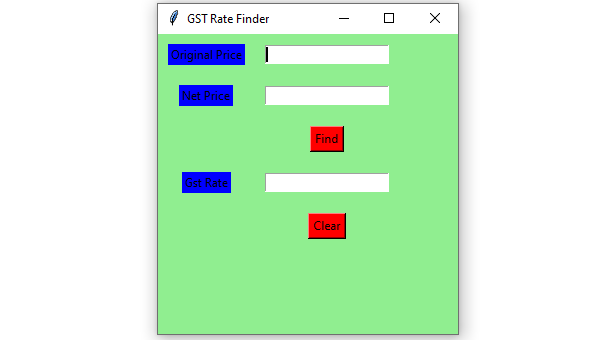# GST Rate Finder GUI using Python-Tkinter

• Last Updated : 15 Jan, 2021

Prerequisites : Introduction to tkinter | Program to calculate GST
Python offers multiple options for developing a GUI (Graphical User Interface). Out of all the GUI methods, Tkinter is the most commonly used method. In this article, we will learn how to create GST Rate Finder GUI application using Tkinter, with a step-by-step guide.

To create a Tkinter:

Attention geek! Strengthen your foundations with the Python Programming Foundation Course and learn the basics.

To begin with, your interview preparations Enhance your Data Structures concepts with the Python DS Course. And to begin with your Machine Learning Journey, join the Machine Learning - Basic Level Course

• Importing the module – tkinter
• Create the main window (container)
• Add any number of widgets to the main window.
• Apply the event Trigger on the widgets.

Below is what the GUI will look like:Let’s create a GUI based GST Rate Finder application :

Below is the implementation :

## Python3

 `# import all functions/classes from the tkinter   ``from` `tkinter ``import` `*`` ` `# Function for finding GST rate``def` `findGst() :`` ` `    ``# take a value from the respective entry boxes``    ``# get method returns current text as string``    ``org_cost``=` `int``(org_priceField.get())``    ` `    ``N_price ``=` `int``(net_priceField.get())`` ` `    ``# calculate GST rate``    ``gst_rate ``=` `((N_price ``-` `org_cost) ``*` `100``) ``/` `org_cost;`` ` `    ``# insert method inserting the  ``    ``# value in the text entry box.``    ``gst_rateField.insert(``10``, ``str``(gst_rate) ``+` `" % "``)``   ` `     ` `# Function for clearing the  ``# contents of all text entry boxes``def` `clearAll():``     ` `     ` `    ``# deleting the content from the entry box``    ``org_priceField.delete(``0``, END)``     ` `    ``net_priceField.delete(``0``, END)``     ` `    ``gst_rateField.delete(``0``, END)``     ` ` ` `# Driver Code``if` `__name__ ``=``=` `"__main__"` `:`` ` `    ``# Create a GUI window``    ``gui ``=` `Tk()``   ` `    ``# Set the background colour of GUI window  ``    ``gui.configure(background ``=` `"light green"``)``   ` `    ``# set the name of tkinter GUI window ``    ``gui.title(``"GST Rate Finder"``)``   ` `    ``# Set the configuration of GUI window``    ``gui.geometry(``"300x300"``)`` ` `    ``# Create a Original Price: label ``    ``org_price ``=` `Label(gui, text ``=` `"Original Price"``,``                      ``bg ``=` `"blue"``)``   ` `    ``# Create a Net Price : label``    ``net_price ``=` `Label(gui, text ``=` `"Net Price"``,``                      ``bg ``=` `"blue"``)`` ` `    ``# Create a Find Button and attached to``    ``# findGst function``    ``find ``=` `Button(gui, text ``=` `"Find"``, fg ``=` `"Black"``,``                  ``bg ``=` `"Red"``,``                  ``command ``=` `findGst)``     ` `    ``# Create a Gst Rate : label ``    ``gst_rate ``=` `Label(gui, text ``=` `"Gst Rate"``, bg ``=` `"blue"``)`` ` `    ``# Create a Clear Button and attached to``    ``# clearAll function``    ``clear ``=` `Button(gui, text ``=` `"Clear"``, fg ``=` `"Black"``,``                   ``bg ``=` `"Red"``,``                   ``command ``=` `clearAll)`` ` `    ``# grid method is used for placing  ``    ``# the widgets at respective positions  ``    ``# in table like structure .`` ` `    ``# padx attributed provide x-axis margin ``    ``# from the root window to the widget.`` ` `    ``# pady attributed provide y-axis``    ``# margin from the widget.``    ``org_price.grid(row ``=` `1``, column ``=` `1``,padx ``=` `10``,pady ``=` `10``)``                  ` `    ``net_price.grid(row ``=` `2``, column ``=` `1``, padx ``=` `10``, pady ``=` `10``)``    ` `    ``find.grid(row ``=` `3``, column ``=` `2``,padx ``=` `10``,pady ``=` `10``)``     ` `    ``gst_rate.grid(row ``=` `4``, column ``=` `1``,padx ``=` `10``, pady ``=` `10``)``     ` `    ``clear.grid(row ``=` `5``, column ``=` `2``, padx ``=` `10``, pady ``=` `10``)`` ` `    ``# Create a text entry box for filling or typing the information.  ``    ``org_priceField ``=` `Entry(gui)``     ` `    ``net_priceField ``=` `Entry(gui)``     ` `    ``gst_rateField ``=` `Entry(gui)`` ` `    ``# grid method is used for placing  ``    ``# the widgets at respective positions  ``    ``# in table like structure .``    ``org_priceField.grid(row ``=` `1``, column ``=` `2` `,padx ``=` `10``,pady ``=` `10``)``     ` `    ``net_priceField.grid(row ``=` `2``, column ``=` `2``, padx ``=` `10``,pady ``=` `10``)``     ` `    ``gst_rateField.grid(row ``=` `4``, column ``=` `2``, padx ``=` `10``,pady ``=` `10``)``     ` `    ``# Start the GUI``    ``gui.mainloop()`

Output :

My Personal Notes arrow_drop_up